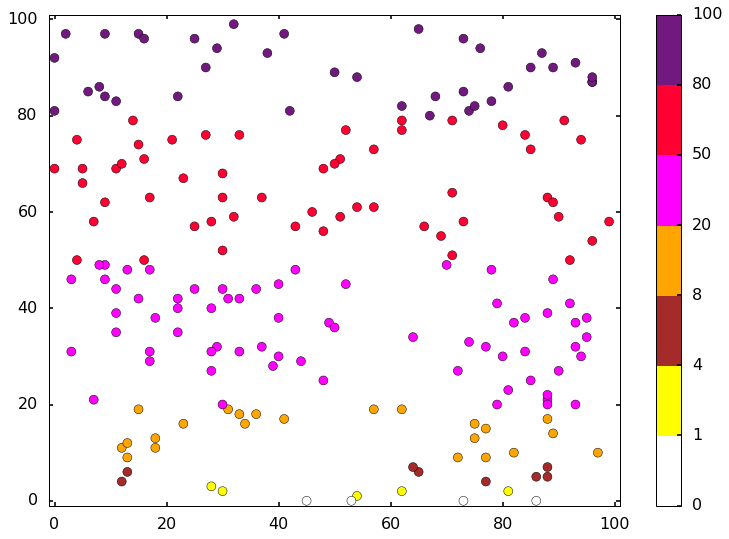## Sunday, December 24, 2017

### Create discrete colormap

Many times, we need different color scales to emphasize different parts. But sometimes, we want to specify some color maps by ourselves, there are ways to do this. Also, sometimes, we want to specify some colors bins, and if my value falls into the bin, I use one specific color. For example, I want the following color schema:
• 0 <= v < 1, white
• 1 <= v < 4, yellow
• 4 <= v < 8, brown
• 8 <= v < 20, orange
• 20 <= v < 50, magenta
• 50 <= v < 80, #FF0033 (sort of red)
• 80 <= v < 100, #721980 (purple)
In the following, I will give an example, you can find the notebook on Qingkai's Github
import numpy as np
import matplotlib.pyplot as plt
import matplotlib.colors as colors
%matplotlib inline
plt.style.use('seaborn-poster')

# generate x and y value between [0, 100)
np.random.seed(10)
x = np.random.randint(low = 0, high=100, size=200)
y = np.random.randint(low = 0, high=100, size=200)

# set colors
colorsList = ['white', 'yellow', 'brown', 'orange', 'magenta', '#FF0033', '#721980']
CustomCmap = colors.ListedColormap(colorsList)
bounds = np.array([0, 1, 4, 8, 20, 50, 80, 100])
norm = colors.BoundaryNorm(boundaries=bounds, ncolors=7)

plt.scatter(x,y,c=y, s = 80, cmap=CustomCmap, norm = norm)
plt.colorbar()
plt.ylim(-1,101)
plt.xlim(-1,101)
plt.show()